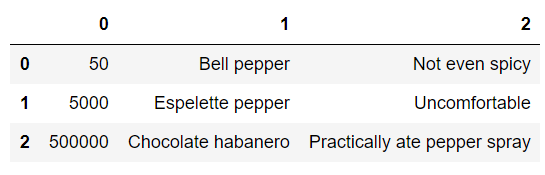# Creating and Manipulating DataFrames in Python with PandasPandas is an open-source Python library for data analysis. It is designed for efficient and intuitive handling and processing of structured data.

#### Introduction

Pandas is an open-source Python library for data analysis. It is designed for efficient and intuitive handling and processing of structured data.

The two main data structures in Pandas are `Series` and `DataFrame``Series` are essentially one-dimensional labeled arrays of any type of data, while `DataFrame`s are two-dimensional, with potentially heterogenous data types, labeled arrays of any type of data. Heterogenous means that not all "rows" need to be of equal size.

In this article we will go through the most common ways of creating a `DataFrame` and methods to change their structure.

We'll be using the Jupyter Notebook since it offers a nice visual representation of `DataFrame`s. Though, any IDE will also do the job, just by calling a `print()` statement on the `DataFrame` object.

#### Creating DataFrames

Whenever you create a `DataFrame`, whether you're creating one manually or generating one from a datasource such as a file - the data has to be ordered in a tabular fashion, as a sequence of rows containing data.

This implies that the rows share the same order of fields, i.e. if you want to have a `DataFrame` with information about a person's name and age, you want to make sure that all your rows hold the information in the same way.

Any discrepancy will cause the `DataFrame` to be faulty, resulting in errors.

##### Creating an Empty DataFrame

To create an empty `DataFrame` is as simple as:

``````import pandas as pd
dataFrame1 = pd.DataFrame()``````

We will take a look at how you can add rows and columns to this empty `DataFrame` while manipulating their structure.

##### Creating a DataFrame From Lists

Following the "sequence of rows with the same order of fields" principle, you can create a `DataFrame` from a list that contains such a sequence, or from multiple lists `zip()`-ed together in such a way that they provide a sequence like that:

``````import pandas as pd

listPepper = [
[50, "Bell pepper", "Not even spicy"],
[5000, "Espelette pepper", "Uncomfortable"],
[500000, "Chocolate habanero", "Practically ate pepper spray"]
]

dataFrame1 = pd.DataFrame(listPepper)

dataFrame1
## If you aren't using Jupyter, you'll have to call `print()`
## print(dataFrame1) ``````

## Fluent Pandas: Pandas Data Structures

Let’s uncover practical details of Pandas’s Series, DataFrame, and Panel. Pandas is a column-oriented data analysis API. It’s a great tool for handling and analyzing input data.

## Basic Data Types in Python | Python Web Development For Beginners

In the programming world, Data types play an important role. Each Variable is stored in different data types and responsible for various functions. Python had two different objects, and They are mutable and immutable objects.

## Data Science With Python Training | Python Data Science Course | Intellipaat

🔵 Intellipaat Data Science with Python course: https://intellipaat.com/python-for-data-science-training/In this Data Science With Python Training video, you...

## Python Pandas Objects - Pandas Series and Pandas Dataframe

In this post, we will learn about pandas’ data structures/objects. Pandas provide two type of data structures:- ### Pandas Series Pandas Series is a one dimensional indexed data, which can hold datatypes like integer, string, boolean, float...

## Python Pandas Tutorial - Data Analysis with Python Pandas

Python Pandas Tutorial will help you get started with Python Pandas Library for various applications including Data analysis. Introduction to Pandas. DataFrames and Series. How To View Data? Selecting Data. Handling Missing Data. Pandas Operations. Merge, Group, Reshape Data. Time Series And Categoricals. Plotting Using Pandas